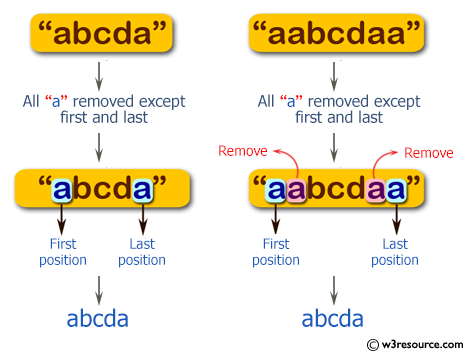﻿ Swift Basic Programming Exercise: Create a new string where all the character "a" have been removed except the first and last positions - w3resource# Swift Basic Programming Exercise: Create a new string where all the character "a" have been removed except the first and last positions

## Swift Basic Programming: Exercise-25 with Solution

Write a Swift program to create a new string where all the character "a" have been removed except the first and last positions.

Pictorial Presentation:Sample Solution:

Swift Code:

``````import Foundation
func string_a(_ input: String) -> String {
var chars = input
let index_start = chars.index(after: chars.startIndex)
let index_end = chars.index(before: chars.endIndex)
let middleRange = index_start ..< index_end

var sub_string = chars.substring(with: middleRange)

while sub_string.characters.contains("a") {
sub_string.remove(at: sub_string.characters.index(of: "a")!)
}

chars.replaceSubrange(middleRange, with: sub_string)

return String(chars)
}

print(string_a("abcd"))
print(string_a("abcda"))
print(string_a("aabcdaa"))
print(string_a("aabaaaacdaa"))
```
```

Sample Output:

```abcd
abcda
abcda
abcda
```

Swift Programming Code Editor:

Improve this sample solution and post your code through Disqus

What is the difficulty level of this exercise?

﻿